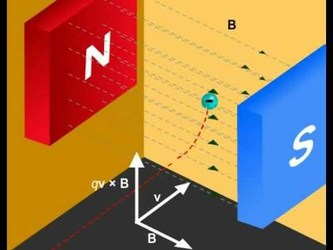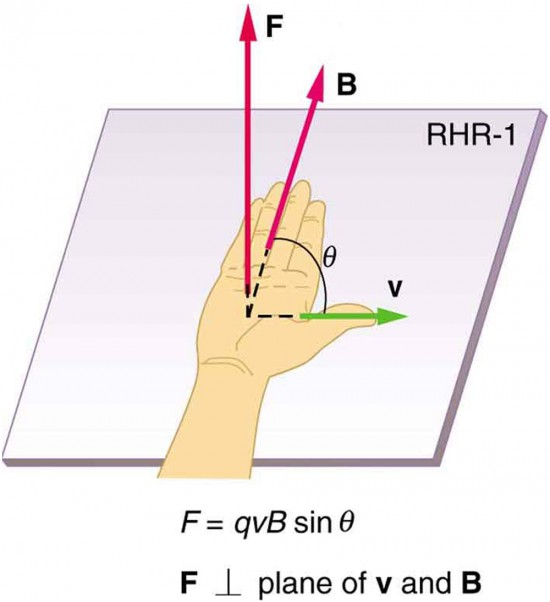Force on Moving Charge in a Magnetic FieldForce on moving charge in a magnetic field

Consider a moving unit positive charge is placed in a magnetic field B with charge +q moving with velocity v making angle θ with the magnetic field.
Here, the charge experiences a force that is perpendicular to the plane of B and v. Experimentally it is found that magnitude of magnetic force F is:1. directly proportional to the magnitude of the charge
∝ q

2. directly proportional to the velocity of the charge
F ∝ v

3. directly proprtional to strength of magnetic field
F ∝ B

4. directly proportional  to sine of angle between v and B
F ∝ sinθ

Combining above equations, we get

F ∝ qvBsinθ
or, F = kqvBsin
θ
where, k is proportionality constant and its value is 1 in SI - units. So, we have force,

F = qvBsinθ

In vector form,

The force is perpendicular toand. This force is called Lorentz magnetic force. We see that F is perpendicular to the plane containing v and B.

Since F acts perpendicular to v and B, so F is a deflecting force. It doesn't change the magnitude of v, but change the direction of motion of the charge when it enters the field B. Since F is perpendicular to displacement of charge, no work is done by F. So, no energy is gained by the charge when it enters the field B and F acts on it.

Reference taken from ' Principle of Physics' - Ayam Publication